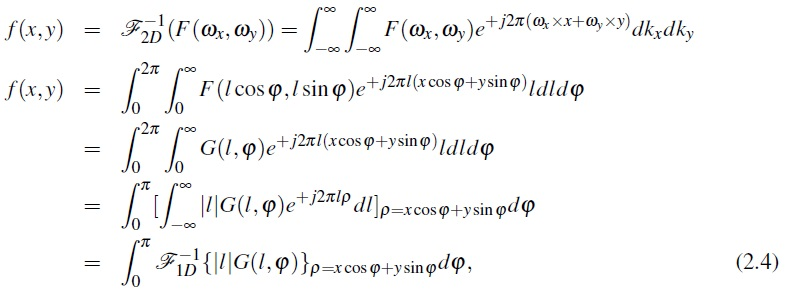To reduce the computational load of back projection (which is of O(N3) for an N N image), Fourier based reconstruction methods have been proposed . The Central Slice Theorem (CST) or Direct Fourier Reconstruction (DFR) relates the 1D Fourier transform of the projections to the 2D Fourier transform of the image, as shown in figure 11. In parallel beam geometry, the 1D Fourier transform of each projection (in the ideal case where the data is noiseless and continuous) is the same as the 2D Fourier coefficients of the object along a line
that passes through the center of the frequency domain with the same angle as the corresponding projection, as can be seen in equation (4). Therefore, this can be used as an alternative to reconstruct the CT images by computing the 1D Fourier coefficients of the projection and putting them along the corresponding line in the 2D Fourier domain. This method requires the interpolation of polar data onto a Cartesian grid followed by an inverse 2D Fourier transform on the same grid to reconstruct the CT image.If the projections were continuous with infinite number of measurements, DFR would be an exact reconstruction algorithm. However, in reality the scan continuity condition is not possible due to a limited number of projections. Thus, to reconstruct a perfect image, the missing coefficients must be interpolated. This is a source for additional artifacts, which needs an over sampled projection to decrease its effect. However, oversampled projections means that the patient is exposed to a higher radiation dose.Figure 11: Central Slice Theorem and polar to Cartesian interpolation.